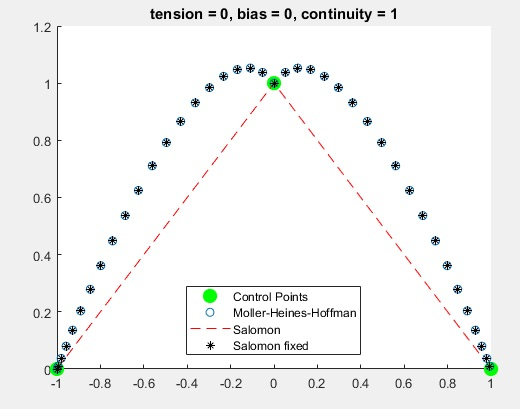Last Updated 19 Nov 2017.

Error found by Chris Wu

Page 281 line 4: should be (-2,-1.5,-1,-0.5,0,0.5,1,1.5) (the value -1 is missing).

------

Error found by Joseph North

Page 449 reference Lagrange (1877):
Le\c cons \'El\'ementaires Sur Les Math\'ematiques, Don\'ees \a l'Ecole Normale en 1795,''
should be
Le\c cons \'El\'ementaires Sur Les Math\'ematiques, Donn\'ees \a l'Ecole Normale en 1795,''

------

Error found by Tom Williams

Page 160: Matrix B in the large display equation in paragraph -3 should include a factor of 1/60.

------------------        -------------------         ----------------

(Apr 2011) Error found by Bill Wilburn

Page 88, Figure 3.4a: The correct coordinates of point (0,0,-0.5) should be (2,1,-0.5).

---------

(Jan 2012) Error found by Pieter Barendrecht

Page 232, end of section 6.21: "0, 0.25, 0.5, 0.75, and 1" should be "0, 0.33, 0.67, and 1". Four, not five, values of $u_k$ are needed because there are 3x4 control points in our example.

Page 261, line 14: "... or order 5, (quintic, with one segment)." Should be "... or order 5, (quartic, with one segment)."

(Feb 2012) Page 253, Equation (7.4). 2b_2+b_1=0 should be 2b_2+b_1=a_1 and 2c_2+c_1=0 should be 2c_2+c_1=b_1.

---------

(Jul 2012) Error found by Aleksy Jones

The expression for $d$ in the middle of page 298, "d=D_3^2/((D_3+D_4+D_5)(D_4+D_5))" should be "d=D_3^2/((D_3+D_4+D_5)(D_3+D_4))".

--------

(Feb 2015) Error found by Ricardo Marroquim

Page 20, second display equation from the top (line approximately -10). The end of the equation should be "T(t) \cdot M \cdot G".

(Mar 2016) Error found by Randy Tarr

Page 278: In the calculation of N_{03}(t) in the interval 1 =< t < 2, the first term should be t/2(2 - t) rather than t^2/2(2 - t).

--------

(Apr 2017) Error found by Rodrigo Silveira

Page 268: The top display equation (which links the cubic B-splines to Bezier curves) is missing a factor of 1/6 in front of the leftmost matrix.

---------

(Nov 2017) Error found by Peeter Parna

Page 169: The definition of the arriving/departing tangents on page 169 is inconsistent with the final definition given on page 171 where the sign of the continuity parameter is swapped. Thus, for example, Equation 5.35 should be

$$\bfP_k^d=\bfP^t_k(0)={{1\over2}} (1-T_k)(1+b_k)(1+c_k)(\bfP_k-\bfP_{k-1})+{{1\over2}} (1-T_k)(1-b_k)(1-c_k)(\bfP_{k+1}-\bfP_k),\eqno{5.35}$$

and similarly for Equation 5.36. This essentially causes the parameter to have the opposite effect. The following figures illustrate the correct effect and serve to correct parts of Figure 5.17.-----------

errata \e'rat-e\ n

a: a list of corrigenda

corrigendum \kor-e'jen-dem\ n

a: an error in a printed work discovered after printing
and shown with its correction on a separate sheet